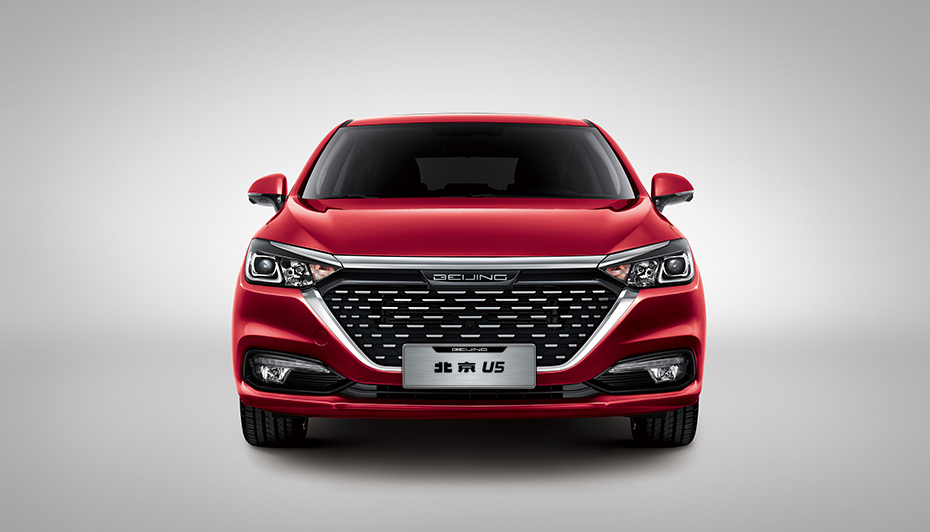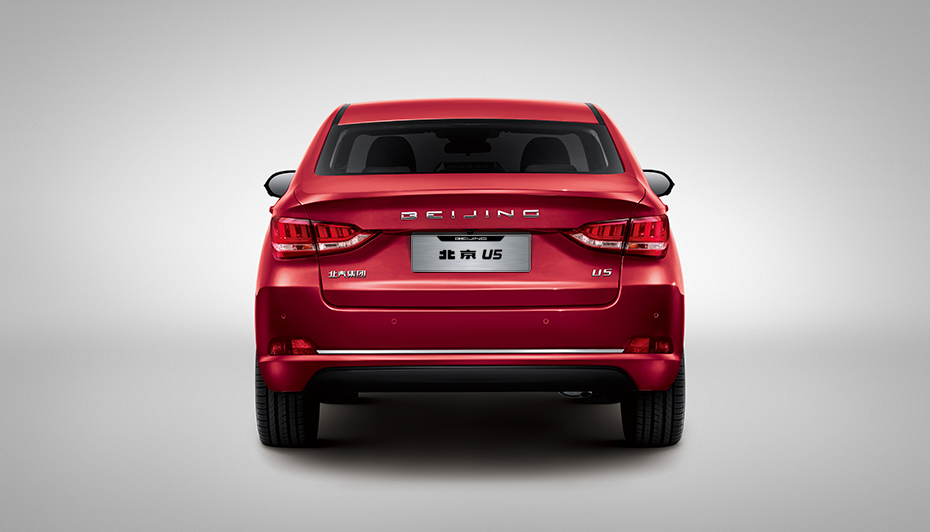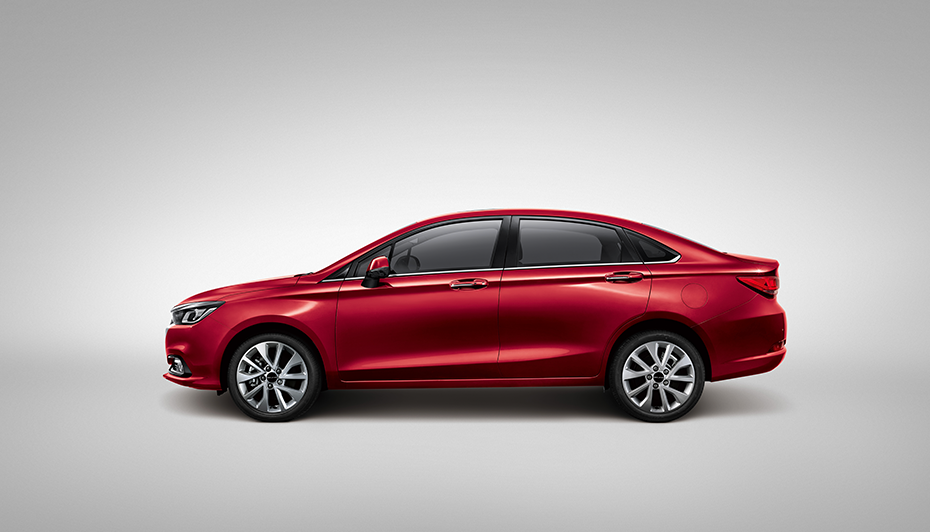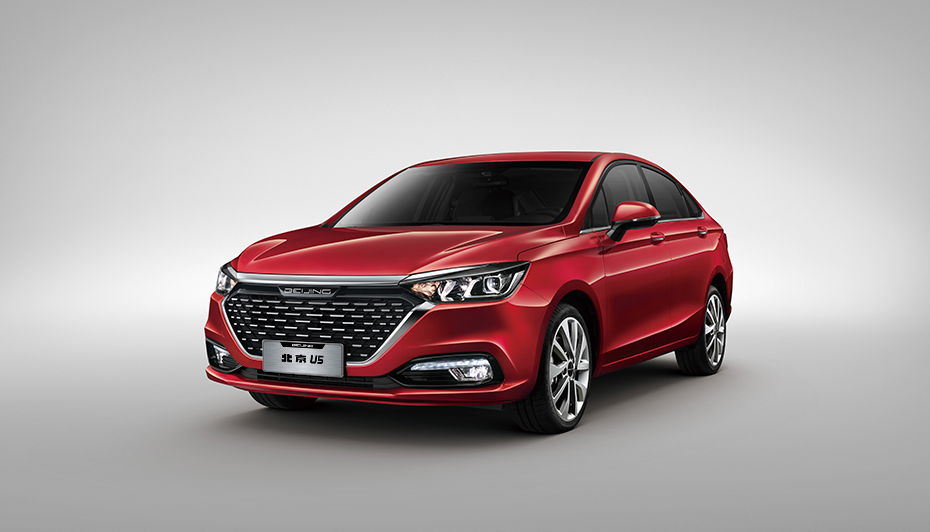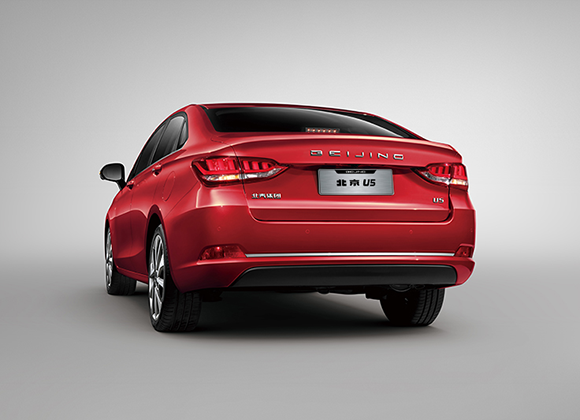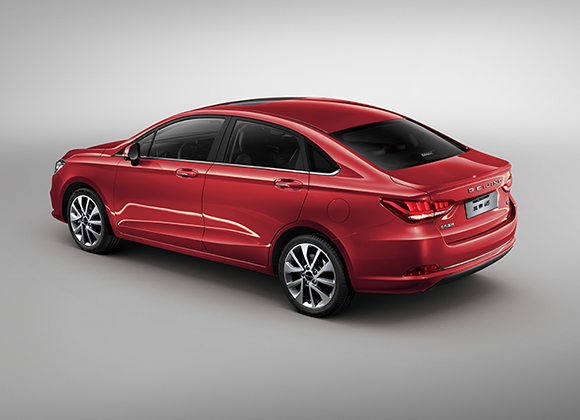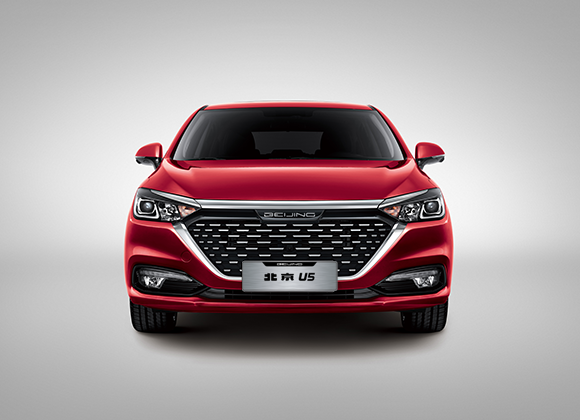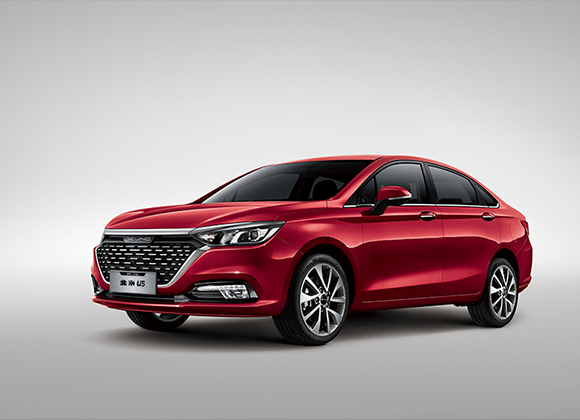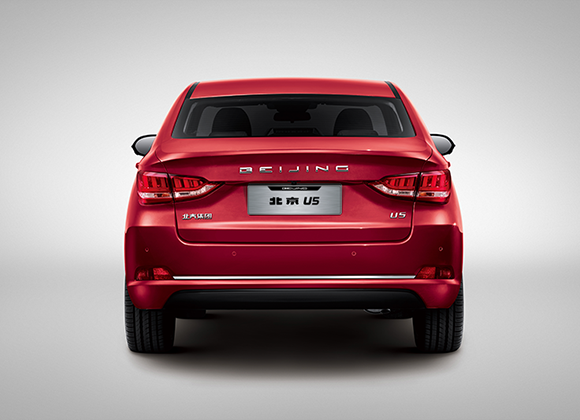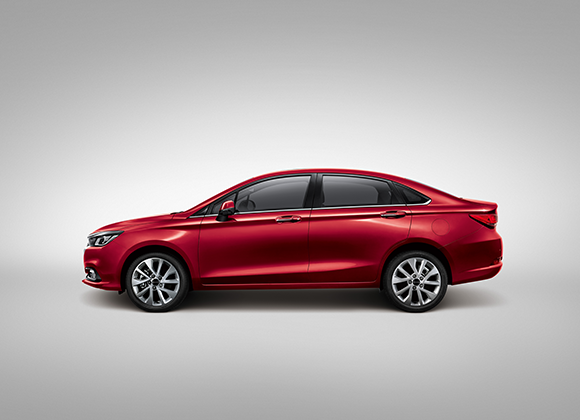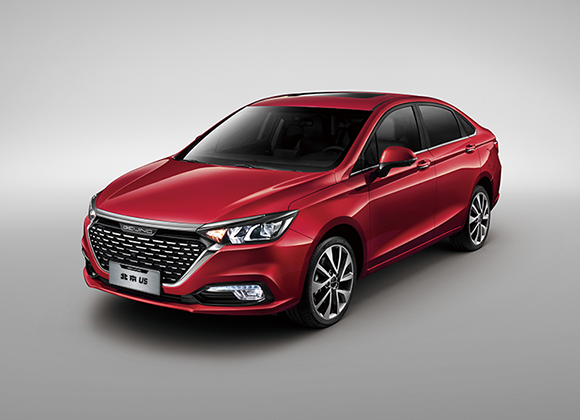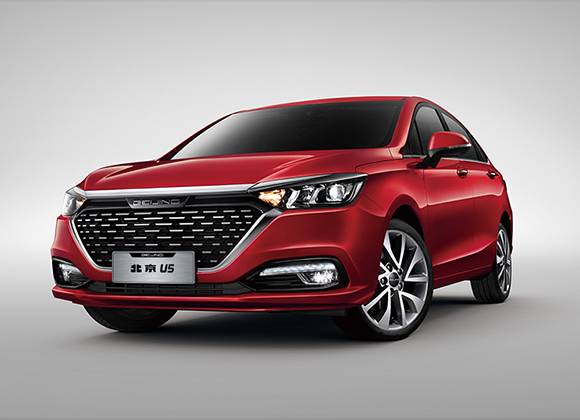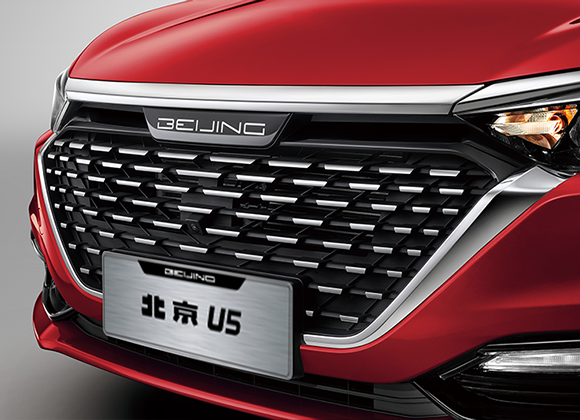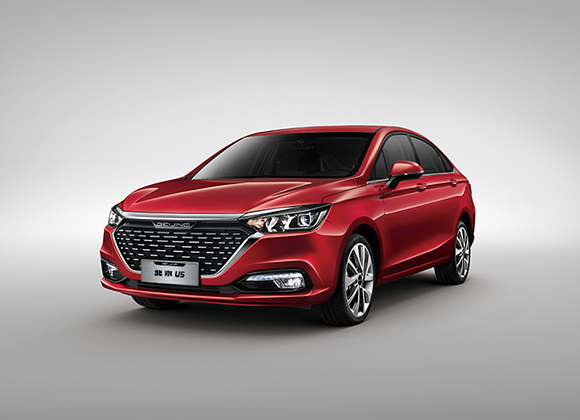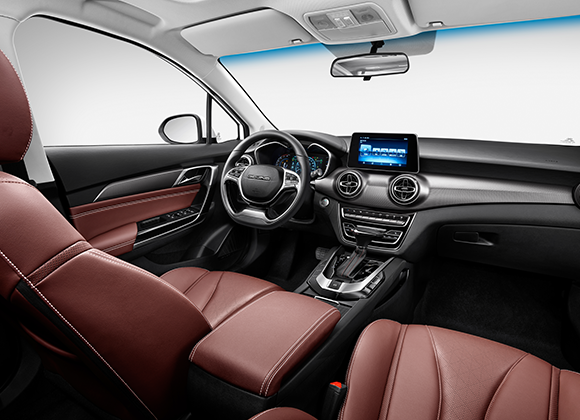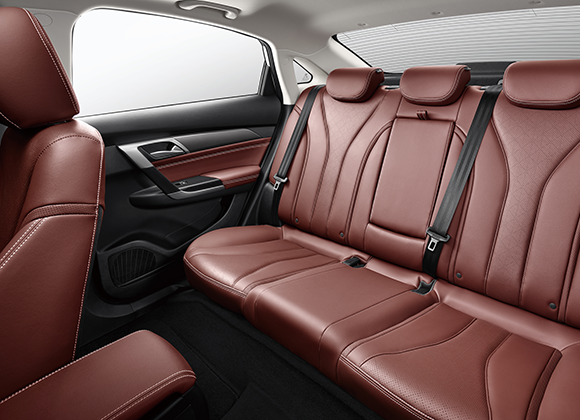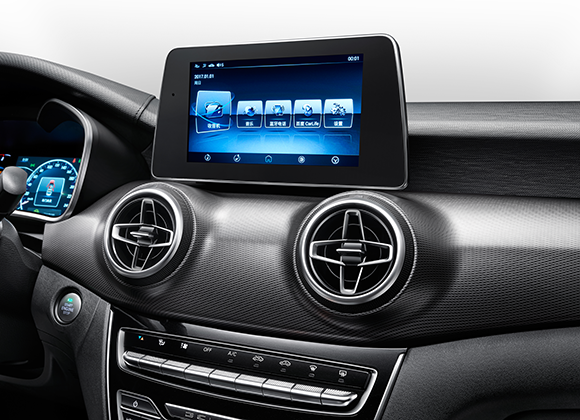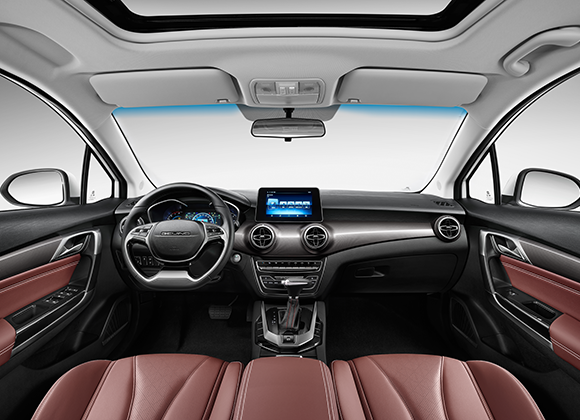• 指导价（元）
• 69900

• 4630
• 1820
• 1480
• 轴距
• 2670
• 驱动
• 前置前驱
• 前悬挂
• 麦弗逊式
• 后悬挂
• H扭力梁
• 前制动器类型
• 通风盘式
• 后制动器类型
• 盘式
• 驻车制动类型
• 手刹
• 轮胎规格
• 205/55R16
• 电动助力转向
• 燃油箱容积（L）
• 48
• 换挡提醒

• 型式
• A151A1
• 最大功率（KW）
• 85
• 最大扭矩（N.m）
• 148
• 变速器
• 5MT
• 排放标准
• 国六

• ABS＋EBD
• ESP+TCS
• AutoHold自动驻车功能
• 上坡辅助
• 液压制动辅助（HBA+HBB)
• 驾驶位气囊
• 副驾驶气囊
• 前排侧安全气囊
• 侧气帘
• 预紧限力式前排座椅三点式安全带
• 后排座椅中间位置三点式安全带
• 安全带高度调节（B柱）
• 驾驶席安全带未系提醒（符号+声音）
• 副驾驶席安全带未系提醒（符号+声音）
• 发动机电子防盗
• 油路自动切断系统
• 防盗报警系统
• 儿童门锁
• ISOFIX固定装置2个
• 行车灯未关报警
• 倒车影像系统
• 定速巡航
• 后泊车雷达
• 车速感应门锁
• 一键启动
• 无钥匙进入
• 智能钥匙 2把
• 中控锁
• 胎压报警
• 行车记录仪
• 空气净化（PM2.5过滤）

• 电动天窗
• 普通白玻
• 普通雨刮（仿无骨）
• 雨量感应雨刮
• 后风挡玻璃除霜
• 外后视镜电动折叠
• 电动调节外后视镜
• 电加热外后视镜
• 外后视镜带转向灯
• 前车门电动车窗
• 后车门电动车窗
• 四门车窗一键降
• 16寸铝合金轮辋
• 17寸铝合金轮毂
• 非全尺寸备胎
• 印刷天线
• 发动机装饰罩
• 机舱全覆盖

• PU 方向盘
• 皮质方向盘
• 真皮方向盘
• 方向盘上下可调
• 行车电脑
• 7寸液晶仪表
• 12.3寸液晶仪表
• 手动防眩目内后视镜
• 驾驶员遮阳板带化妆镜
• 副驾驶遮阳板带化妆镜
• 前排中央扶手
• 高CONSOLE

• 皮布双拼座椅
• 皮质座椅
• 前排座椅加热
• 后排座椅头枕
• 3个
• 主驾座椅手动4向调节
• 主驾座椅手动6向调节
• 主驾座椅电动6向调节
• 副驾驶座椅手动4向调节
• 后排座椅整体折叠
• 第二排座椅中央扶手（带杯托）

• 12伏电源
• 标准USB接口带充电功能
• 收音机功能
• 8寸大屏
• 百度 Car Life手机互联
• 9吋大屏（带4G）
• GPS导航
• T-BOX
• 多功能方向盘
• 扬声器
• 2
• 速度感知声音补偿系统
• 蓝牙功能
• 智能车机2.0

• 卤素前大灯
• LED前大灯
• LED日间行车灯
• LED尾灯
• 大灯高度可调
• 自动后行李厢灯
• 弯道辅助照明
• 高位刹车灯
• 紧急制动双闪
• 前顶阅读灯（带延时功能）
• 前雾灯
• 后雾灯
• 自动大灯
• 车内氛围灯

• 电动空调
• 自动空调
• 空调带花粉过滤功能

• 珠光白
• 极光银
• 铂金灰
• 冰晶蓝
• 卡门红
• 墨玉黑

• 黑+红内饰（PVC+织物）
• 黑+红棕内饰（超纤+PVC）
• 纯黑内饰（超纤+PVC）
• 指导价（元）
• 76900

• 4630
• 1820
• 1480
• 轴距
• 2670
• 驱动
• 前置前驱
• 前悬挂
• 麦弗逊式
• 后悬挂
• H扭力梁
• 前制动器类型
• 通风盘式
• 后制动器类型
• 盘式
• 驻车制动类型
• EPB
• 轮胎规格
• 205/55R16
• 电动助力转向
• 燃油箱容积（L）
• 48
• 换挡提醒

• 型式
• A151A1
• 最大功率（KW）
• 85
• 最大扭矩（N.m）
• 148
• 变速器
• 5MT
• 排放标准
• 国六

• ABS＋EBD
• ESP+TCS
• AutoHold自动驻车功能
• 上坡辅助
• 液压制动辅助（HBA+HBB)
• 驾驶位气囊
• 副驾驶气囊
• 前排侧安全气囊
• 侧气帘
• 预紧限力式前排座椅三点式安全带
• 后排座椅中间位置三点式安全带
• 安全带高度调节（B柱）
• 驾驶席安全带未系提醒（符号+声音）
• 副驾驶席安全带未系提醒（符号+声音）
• 发动机电子防盗
• 油路自动切断系统
• 防盗报警系统
• 儿童门锁
• ISOFIX固定装置2个
• 行车灯未关报警
• 倒车影像系统
• 定速巡航
• 后泊车雷达
• 车速感应门锁
• 一键启动
• 无钥匙进入
• 智能钥匙 2把
• 中控锁
• 胎压报警
• 行车记录仪
• 空气净化（PM2.5过滤）

• 电动天窗
• 普通白玻
• 普通雨刮（仿无骨）
• 雨量感应雨刮
• 后风挡玻璃除霜
• 外后视镜电动折叠
• 电动调节外后视镜
• 电加热外后视镜
• 外后视镜带转向灯
• 前车门电动车窗
• 后车门电动车窗
• 四门车窗一键降
• 16寸铝合金轮辋
• 17寸铝合金轮毂
• 非全尺寸备胎
• 印刷天线
• 发动机装饰罩
• 机舱全覆盖

• PU 方向盘
• 皮质方向盘
• 真皮方向盘
• 方向盘上下可调
• 行车电脑
• 7寸液晶仪表
• 12.3寸液晶仪表
• 手动防眩目内后视镜
• 驾驶员遮阳板带化妆镜
• 副驾驶遮阳板带化妆镜
• 前排中央扶手
• 高CONSOLE

• 皮布双拼座椅
• 皮质座椅
• 前排座椅加热
• 后排座椅头枕
• 3个
• 主驾座椅手动4向调节
• 主驾座椅手动6向调节
• 主驾座椅电动6向调节
• 副驾驶座椅手动4向调节
• 后排座椅整体折叠
• 第二排座椅中央扶手（带杯托）

• 12伏电源
• 标准USB接口带充电功能
• 收音机功能
• 8寸大屏
• 百度 Car Life手机互联
• 9吋大屏（带4G）
• GPS导航
• T-BOX
• 多功能方向盘
• 扬声器
• 6
• 速度感知声音补偿系统
• 蓝牙功能
• 智能车机2.0

• 卤素前大灯
• LED前大灯
• LED日间行车灯
• LED尾灯
• 大灯高度可调
• 自动后行李厢灯
• 弯道辅助照明
• 高位刹车灯
• 紧急制动双闪
• 前顶阅读灯（带延时功能）
• 前雾灯
• 后雾灯
• 自动大灯
• 车内氛围灯

• 电动空调
• 自动空调
• 空调带花粉过滤功能

• 珠光白
• 极光银
• 铂金灰
• 冰晶蓝
• 卡门红
• 墨玉黑

• 黑+红内饰（PVC+织物）
• 黑+红棕内饰（超纤+PVC）
• 纯黑内饰（超纤+PVC）
• 指导价（元）
• 79900

• 4630
• 1820
• 1480
• 轴距
• 2670
• 驱动
• 前置前驱
• 前悬挂
• 麦弗逊式
• 后悬挂
• H扭力梁
• 前制动器类型
• 通风盘式
• 后制动器类型
• 盘式
• 驻车制动类型
• EPB
• 轮胎规格
• 205/55R16
• 电动助力转向
• 燃油箱容积（L）
• 48
• 换挡提醒

• 型式
• A151A1
• 最大功率（KW）
• 77
• 最大扭矩（N.m）
• 134
• 变速器
• CVT
• 排放标准
• 国六

• ABS＋EBD
• ESP+TCS
• AutoHold自动驻车功能
• 上坡辅助
• 液压制动辅助（HBA+HBB)
• 驾驶位气囊
• 副驾驶气囊
• 前排侧安全气囊
• 侧气帘
• 预紧限力式前排座椅三点式安全带
• 后排座椅中间位置三点式安全带
• 安全带高度调节（B柱）
• 驾驶席安全带未系提醒（符号+声音）
• 副驾驶席安全带未系提醒（符号+声音）
• 发动机电子防盗
• 油路自动切断系统
• 防盗报警系统
• 儿童门锁
• ISOFIX固定装置2个
• 行车灯未关报警
• 倒车影像系统
• 定速巡航
• 后泊车雷达
• 车速感应门锁
• 一键启动
• 无钥匙进入
• 智能钥匙 2把
• 中控锁
• 胎压报警
• 行车记录仪
• 空气净化（PM2.5过滤）

• 电动天窗
• 普通白玻
• 普通雨刮（仿无骨）
• 雨量感应雨刮
• 后风挡玻璃除霜
• 外后视镜电动折叠
• 电动调节外后视镜
• 电加热外后视镜
• 外后视镜带转向灯
• 前车门电动车窗
• 后车门电动车窗
• 四门车窗一键降
• 16寸铝合金轮辋
• 17寸铝合金轮毂
• 非全尺寸备胎
• 印刷天线
• 发动机装饰罩
• 机舱全覆盖

• PU 方向盘
• 皮质方向盘
• 真皮方向盘
• 方向盘上下可调
• 行车电脑
• 7寸液晶仪表
• 12.3寸液晶仪表
• 手动防眩目内后视镜
• 驾驶员遮阳板带化妆镜
• 副驾驶遮阳板带化妆镜
• 前排中央扶手
• 高CONSOLE

• 皮布双拼座椅
• 皮质座椅
• 前排座椅加热
• 后排座椅头枕
• 3个
• 主驾座椅手动4向调节
• 主驾座椅手动6向调节
• 主驾座椅电动6向调节
• 副驾驶座椅手动4向调节
• 后排座椅整体折叠
• 第二排座椅中央扶手（带杯托）

• 12伏电源
• 标准USB接口带充电功能
• 收音机功能
• 8寸大屏
• 百度 Car Life手机互联
• 9吋大屏（带4G）
• GPS导航
• T-BOX
• 多功能方向盘
• 扬声器
• 2
• 速度感知声音补偿系统
• 蓝牙功能
• 智能车机2.0

• 卤素前大灯
• LED前大灯
• LED日间行车灯
• LED尾灯
• 大灯高度可调
• 自动后行李厢灯
• 弯道辅助照明
• 高位刹车灯
• 紧急制动双闪
• 前顶阅读灯（带延时功能）
• 前雾灯
• 后雾灯
• 自动大灯
• 车内氛围灯

• 电动空调
• 自动空调
• 空调带花粉过滤功能

• 珠光白
• 极光银
• 铂金灰
• 冰晶蓝
• 卡门红
• 墨玉黑

• 黑+红内饰（PVC+织物）
• 黑+红棕内饰（超纤+PVC）
• 纯黑内饰（超纤+PVC）
• 指导价（元）
• 86900

• 4630
• 1820
• 1480
• 轴距
• 2670
• 驱动
• 前置前驱
• 前悬挂
• 麦弗逊式
• 后悬挂
• H扭力梁
• 前制动器类型
• 通风盘式
• 后制动器类型
• 盘式
• 驻车制动类型
• EPB
• 轮胎规格
• 205/55R16
• 电动助力转向
• 燃油箱容积（L）
• 48
• 换挡提醒

• 型式
• A151A1
• 最大功率（KW）
• 77
• 最大扭矩（N.m）
• 134
• 变速器
• CVT
• 排放标准
• 国六

• ABS＋EBD
• ESP+TCS
• AutoHold自动驻车功能
• 上坡辅助
• 液压制动辅助（HBA+HBB)
• 驾驶位气囊
• 副驾驶气囊
• 前排侧安全气囊
• 侧气帘
• 预紧限力式前排座椅三点式安全带
• 后排座椅中间位置三点式安全带
• 安全带高度调节（B柱）
• 驾驶席安全带未系提醒（符号+声音）
• 副驾驶席安全带未系提醒（符号+声音）
• 发动机电子防盗
• 油路自动切断系统
• 防盗报警系统
• 儿童门锁
• ISOFIX固定装置2个
• 行车灯未关报警
• 倒车影像系统
• 定速巡航
• 后泊车雷达
• 车速感应门锁
• 一键启动
• 无钥匙进入
• 智能钥匙 2把
• 中控锁
• 胎压报警
• 行车记录仪
• 空气净化（PM2.5过滤）

• 电动天窗
• 普通白玻
• 普通雨刮（仿无骨）
• 雨量感应雨刮
• 后风挡玻璃除霜
• 外后视镜电动折叠
• 电动调节外后视镜
• 电加热外后视镜
• 外后视镜带转向灯
• 前车门电动车窗
• 后车门电动车窗
• 四门车窗一键降
• 16寸铝合金轮辋
• 17寸铝合金轮毂
• 非全尺寸备胎
• 印刷天线
• 发动机装饰罩
• 机舱全覆盖

• PU 方向盘
• 皮质方向盘
• 真皮方向盘
• 方向盘上下可调
• 行车电脑
• 7寸液晶仪表
• 12.3寸液晶仪表
• 手动防眩目内后视镜
• 驾驶员遮阳板带化妆镜
• 副驾驶遮阳板带化妆镜
• 前排中央扶手
• 高CONSOLE

• 皮布双拼座椅
• 皮质座椅
• 前排座椅加热
• 后排座椅头枕
• 3个
• 主驾座椅手动4向调节
• 主驾座椅手动6向调节
• 主驾座椅电动6向调节
• 副驾驶座椅手动4向调节
• 后排座椅整体折叠
• 第二排座椅中央扶手（带杯托）

• 12伏电源
• 标准USB接口带充电功能
• 收音机功能
• 8寸大屏
• 百度 Car Life手机互联
• 9吋大屏（带4G）
• GPS导航
• T-BOX
• 多功能方向盘
• 扬声器
• 6
• 速度感知声音补偿系统
• 蓝牙功能
• 智能车机2.0

• 卤素前大灯
• LED前大灯
• LED日间行车灯
• LED尾灯
• 大灯高度可调
• 自动后行李厢灯
• 弯道辅助照明
• 高位刹车灯
• 紧急制动双闪
• 前顶阅读灯（带延时功能）
• 前雾灯
• 后雾灯
• 自动大灯
• 车内氛围灯

• 电动空调
• 自动空调
• 空调带花粉过滤功能

• 珠光白
• 极光银
• 铂金灰
• 冰晶蓝
• 卡门红
• 墨玉黑

• 黑+红内饰（PVC+织物）
• 黑+红棕内饰（超纤+PVC）
• 纯黑内饰（超纤+PVC）
• 指导价（元）
• 76900

• 4630
• 1820
• 1480
• 轴距
• 2670
• 驱动
• 前置前驱
• 前悬挂
• 麦弗逊式
• 后悬挂
• H扭力梁
• 前制动器类型
• 通风盘式
• 后制动器类型
• 盘式
• 驻车制动类型
• EPB
• 轮胎规格
• 205/55R16
• 电动助力转向
• 燃油箱容积（L）
• 48
• 换挡提醒

• 型式
• A151R1
• 最大功率（KW）
• 110
• 最大扭矩（N.m）
• 210
• 变速器
• 6MT
• 排放标准
• 国六

• ABS＋EBD
• ESP+TCS
• AutoHold自动驻车功能
• 上坡辅助
• 液压制动辅助（HBA+HBB)
• 驾驶位气囊
• 副驾驶气囊
• 前排侧安全气囊
• 侧气帘
• 预紧限力式前排座椅三点式安全带
• 后排座椅中间位置三点式安全带
• 安全带高度调节（B柱）
• 驾驶席安全带未系提醒（符号+声音）
• 副驾驶席安全带未系提醒（符号+声音）
• 发动机电子防盗
• 油路自动切断系统
• 防盗报警系统
• 儿童门锁
• ISOFIX固定装置2个
• 行车灯未关报警
• 倒车影像系统
• 定速巡航
• 后泊车雷达
• 车速感应门锁
• 一键启动
• 无钥匙进入
• 智能钥匙 2把
• 中控锁
• 胎压报警
• 行车记录仪
• 空气净化（PM2.5过滤）

• 电动天窗
• 普通白玻
• 普通雨刮（仿无骨）
• 雨量感应雨刮
• 后风挡玻璃除霜
• 外后视镜电动折叠
• 电动调节外后视镜
• 电加热外后视镜
• 外后视镜带转向灯
• 前车门电动车窗
• 后车门电动车窗
• 四门车窗一键降
• 16寸铝合金轮辋
• 17寸铝合金轮毂
• 非全尺寸备胎
• 印刷天线
• 发动机装饰罩
• 机舱全覆盖

• PU 方向盘
• 皮质方向盘
• 真皮方向盘
• 方向盘上下可调
• 行车电脑
• 7寸液晶仪表
• 12.3寸液晶仪表
• 手动防眩目内后视镜
• 驾驶员遮阳板带化妆镜
• 副驾驶遮阳板带化妆镜
• 前排中央扶手
• 高CONSOLE

• 皮布双拼座椅
• 皮质座椅
• 前排座椅加热
• 后排座椅头枕
• 3个
• 主驾座椅手动4向调节
• 主驾座椅手动6向调节
• 主驾座椅电动6向调节
• 副驾驶座椅手动4向调节
• 后排座椅整体折叠
• 第二排座椅中央扶手（带杯托）

• 12伏电源
• 标准USB接口带充电功能
• 收音机功能
• 8寸大屏
• 百度 Car Life手机互联
• 9吋大屏（带4G）
• GPS导航
• T-BOX
• 多功能方向盘
• 扬声器
• 6
• 速度感知声音补偿系统
• 蓝牙功能
• 智能车机2.0

• 卤素前大灯
• LED前大灯
• LED日间行车灯
• LED尾灯
• 大灯高度可调
• 自动后行李厢灯
• 弯道辅助照明
• 高位刹车灯
• 紧急制动双闪
• 前顶阅读灯（带延时功能）
• 前雾灯
• 后雾灯
• 自动大灯
• 车内氛围灯

• 电动空调
• 自动空调
• 空调带花粉过滤功能

• 珠光白
• 极光银
• 铂金灰
• 冰晶蓝
• 卡门红
• 墨玉黑

• 黑+红内饰（PVC+织物）
• 黑+红棕内饰（超纤+PVC）
• 纯黑内饰（超纤+PVC）
• 指导价（元）
• 86900

• 4630
• 1820
• 1480
• 轴距
• 2670
• 驱动
• 前置前驱
• 前悬挂
• 麦弗逊式
• 后悬挂
• H扭力梁
• 前制动器类型
• 通风盘式
• 后制动器类型
• 盘式
• 驻车制动类型
• EPB
• 轮胎规格
• 205/55R16
• 电动助力转向
• 燃油箱容积（L）
• 48
• 换挡提醒

• 型式
• A151R1
• 最大功率（KW）
• 110
• 最大扭矩（N.m）
• 210
• 变速器
• CVT
• 排放标准
• 国六

• ABS＋EBD
• ESP+TCS
• AutoHold自动驻车功能
• 上坡辅助
• 液压制动辅助（HBA+HBB)
• 驾驶位气囊
• 副驾驶气囊
• 前排侧安全气囊
• 侧气帘
• 预紧限力式前排座椅三点式安全带
• 后排座椅中间位置三点式安全带
• 安全带高度调节（B柱）
• 驾驶席安全带未系提醒（符号+声音）
• 副驾驶席安全带未系提醒（符号+声音）
• 发动机电子防盗
• 油路自动切断系统
• 防盗报警系统
• 儿童门锁
• ISOFIX固定装置2个
• 行车灯未关报警
• 倒车影像系统
• 定速巡航
• 后泊车雷达
• 车速感应门锁
• 一键启动
• 无钥匙进入
• 智能钥匙 2把
• 中控锁
• 胎压报警
• 行车记录仪
• 空气净化（PM2.5过滤）

• 电动天窗
• 普通白玻
• 普通雨刮（仿无骨）
• 雨量感应雨刮
• 后风挡玻璃除霜
• 外后视镜电动折叠
• 电动调节外后视镜
• 电加热外后视镜
• 外后视镜带转向灯
• 前车门电动车窗
• 后车门电动车窗
• 四门车窗一键降
• 16寸铝合金轮辋
• 17寸铝合金轮毂
• 非全尺寸备胎
• 印刷天线
• 发动机装饰罩
• 机舱全覆盖

• PU 方向盘
• 皮质方向盘
• 真皮方向盘
• 方向盘上下可调
• 行车电脑
• 7寸液晶仪表
• 12.3寸液晶仪表
• 手动防眩目内后视镜
• 驾驶员遮阳板带化妆镜
• 副驾驶遮阳板带化妆镜
• 前排中央扶手
• 高CONSOLE

• 皮布双拼座椅
• 皮质座椅
• 前排座椅加热
• 后排座椅头枕
• 3个
• 主驾座椅手动4向调节
• 主驾座椅手动6向调节
• 主驾座椅电动6向调节
• 副驾驶座椅手动4向调节
• 后排座椅整体折叠
• 第二排座椅中央扶手（带杯托）

• 12伏电源
• 标准USB接口带充电功能
• 收音机功能
• 8寸大屏
• 百度 Car Life手机互联
• 9吋大屏（带4G）
• GPS导航
• T-BOX
• 多功能方向盘
• 扬声器
• 6
• 速度感知声音补偿系统
• 蓝牙功能
• 智能车机2.0

• 卤素前大灯
• LED前大灯
• LED日间行车灯
• LED尾灯
• 大灯高度可调
• 自动后行李厢灯
• 弯道辅助照明
• 高位刹车灯
• 紧急制动双闪
• 前顶阅读灯（带延时功能）
• 前雾灯
• 后雾灯
• 自动大灯
• 车内氛围灯

• 电动空调
• 自动空调
• 空调带花粉过滤功能

• 珠光白
• 极光银
• 铂金灰
• 冰晶蓝
• 卡门红
• 墨玉黑

• 黑+红内饰（PVC+织物）
• 黑+红棕内饰（超纤+PVC）
• 纯黑内饰（超纤+PVC）
• 指导价（元）
• 91900

• 4630
• 1820
• 1480
• 轴距
• 2670
• 驱动
• 前置前驱
• 前悬挂
• 麦弗逊式
• 后悬挂
• H扭力梁
• 前制动器类型
• 通风盘式
• 后制动器类型
• 盘式
• 驻车制动类型
• EPB
• 轮胎规格
• 205/55R16
• 电动助力转向
• 燃油箱容积（L）
• 48
• 换挡提醒

• 型式
• A151R1
• 最大功率（KW）
• 110
• 最大扭矩（N.m）
• 210
• 变速器
• CVT
• 排放标准
• 国六

• ABS＋EBD
• ESP+TCS
• AutoHold自动驻车功能
• 上坡辅助
• 液压制动辅助（HBA+HBB)
• 驾驶位气囊
• 副驾驶气囊
• 前排侧安全气囊
• 侧气帘
• 预紧限力式前排座椅三点式安全带
• 后排座椅中间位置三点式安全带
• 安全带高度调节（B柱）
• 驾驶席安全带未系提醒（符号+声音）
• 副驾驶席安全带未系提醒（符号+声音）
• 发动机电子防盗
• 油路自动切断系统
• 防盗报警系统
• 儿童门锁
• ISOFIX固定装置2个
• 行车灯未关报警
• 倒车影像系统
• 定速巡航
• 后泊车雷达
• 车速感应门锁
• 一键启动
• 无钥匙进入
• 智能钥匙 2把
• 中控锁
• 胎压报警
• 行车记录仪
• 空气净化（PM2.5过滤）

• 电动天窗
• 普通白玻
• 普通雨刮（仿无骨）
• 雨量感应雨刮
• 后风挡玻璃除霜
• 外后视镜电动折叠
• 电动调节外后视镜
• 电加热外后视镜
• 外后视镜带转向灯
• 前车门电动车窗
• 后车门电动车窗
• 四门车窗一键降
• 16寸铝合金轮辋
• 17寸铝合金轮毂
• 非全尺寸备胎
• 印刷天线
• 发动机装饰罩
• 机舱全覆盖

• PU 方向盘
• 皮质方向盘
• 真皮方向盘
• 方向盘上下可调
• 行车电脑
• 7寸液晶仪表
• 12.3寸液晶仪表
• 手动防眩目内后视镜
• 驾驶员遮阳板带化妆镜
• 副驾驶遮阳板带化妆镜
• 前排中央扶手
• 高CONSOLE

• 皮布双拼座椅
• 皮质座椅
• 前排座椅加热
• 后排座椅头枕
• 3个
• 主驾座椅手动4向调节
• 主驾座椅手动6向调节
• 主驾座椅电动6向调节
• 副驾驶座椅手动4向调节
• 后排座椅整体折叠
• 第二排座椅中央扶手（带杯托）

• 12伏电源
• 标准USB接口带充电功能
• 收音机功能
• 8寸大屏
• 百度 Car Life手机互联
• 9吋大屏（带4G）
• GPS导航
• T-BOX
• 多功能方向盘
• 扬声器
• 6
• 速度感知声音补偿系统
• 蓝牙功能
• 智能车机2.0

• 卤素前大灯
• LED前大灯
• LED日间行车灯
• LED尾灯
• 大灯高度可调
• 自动后行李厢灯
• 弯道辅助照明
• 高位刹车灯
• 紧急制动双闪
• 前顶阅读灯（带延时功能）
• 前雾灯
• 后雾灯
• 自动大灯
• 车内氛围灯

• 电动空调
• 自动空调
• 空调带花粉过滤功能

• 珠光白
• 极光银
• 铂金灰
• 冰晶蓝
• 卡门红
• 墨玉黑

• 黑+红内饰（PVC+织物）
• 黑+红棕内饰（超纤+PVC）
• 纯黑内饰（超纤+PVC）
• 指导价（元）
• 99900

• 4630
• 1820
• 1480
• 轴距
• 2670
• 驱动
• 前置前驱
• 前悬挂
• 麦弗逊式
• 后悬挂
• H扭力梁
• 前制动器类型
• 通风盘式
• 后制动器类型
• 盘式
• 驻车制动类型
• EPB
• 轮胎规格
• 205/50R17
• 电动助力转向
• 燃油箱容积（L）
• 48
• 换挡提醒

• 型式
• A151R1
• 最大功率（KW）
• 110
• 最大扭矩（N.m）
• 210
• 变速器
• CVT
• 排放标准
• 国六

• ABS＋EBD
• ESP+TCS
• AutoHold自动驻车功能
• 上坡辅助
• 液压制动辅助（HBA+HBB)
• 驾驶位气囊
• 副驾驶气囊
• 前排侧安全气囊
• 侧气帘
• 预紧限力式前排座椅三点式安全带
• 后排座椅中间位置三点式安全带
• 安全带高度调节（B柱）
• 驾驶席安全带未系提醒（符号+声音）
• 副驾驶席安全带未系提醒（符号+声音）
• 发动机电子防盗
• 油路自动切断系统
• 防盗报警系统
• 儿童门锁
• ISOFIX固定装置2个
• 行车灯未关报警
• 倒车影像系统
• 定速巡航
• 后泊车雷达
• 车速感应门锁
• 一键启动
• 无钥匙进入
• 智能钥匙 2把
• 中控锁
• 胎压报警
• 行车记录仪
• 空气净化（PM2.5过滤）

• 电动天窗
• 普通白玻
• 普通雨刮（仿无骨）
• 雨量感应雨刮
• 后风挡玻璃除霜
• 外后视镜电动折叠
• 电动调节外后视镜
• 电加热外后视镜
• 外后视镜带转向灯
• 前车门电动车窗
• 后车门电动车窗
• 四门车窗一键降
• 16寸铝合金轮辋
• 17寸铝合金轮毂
• 非全尺寸备胎
• 印刷天线
• 发动机装饰罩
• 机舱全覆盖

• PU 方向盘
• 皮质方向盘
• 真皮方向盘
• 方向盘上下可调
• 行车电脑
• 7寸液晶仪表
• 12.3寸液晶仪表
• 手动防眩目内后视镜
• 驾驶员遮阳板带化妆镜
• 副驾驶遮阳板带化妆镜
• 前排中央扶手
• 高CONSOLE

• 皮布双拼座椅
• 皮质座椅
• 前排座椅加热
• 后排座椅头枕
• 3个
• 主驾座椅手动4向调节
• 主驾座椅手动6向调节
• 主驾座椅电动6向调节
• 副驾驶座椅手动4向调节
• 后排座椅整体折叠
• 第二排座椅中央扶手（带杯托）

• 12伏电源
• 标准USB接口带充电功能
• 收音机功能
• 8寸大屏
• 百度 Car Life手机互联
• 9吋大屏（带4G）
• GPS导航
• T-BOX
• 多功能方向盘
• 扬声器
• 6
• 速度感知声音补偿系统
• 蓝牙功能
• 智能车机2.0

• 卤素前大灯
• LED前大灯
• LED日间行车灯
• LED尾灯
• 大灯高度可调
• 自动后行李厢灯
• 弯道辅助照明
• 高位刹车灯
• 紧急制动双闪
• 前顶阅读灯（带延时功能）
• 前雾灯
• 后雾灯
• 自动大灯
• 车内氛围灯

• 电动空调
• 自动空调
• 空调带花粉过滤功能

• 珠光白
• 极光银
• 铂金灰
• 冰晶蓝
• 卡门红
• 墨玉黑

• 黑+红内饰（PVC+织物）
• 黑+红棕内饰（超纤+PVC）
• 纯黑内饰（超纤+PVC）

## 精彩集锦

图集